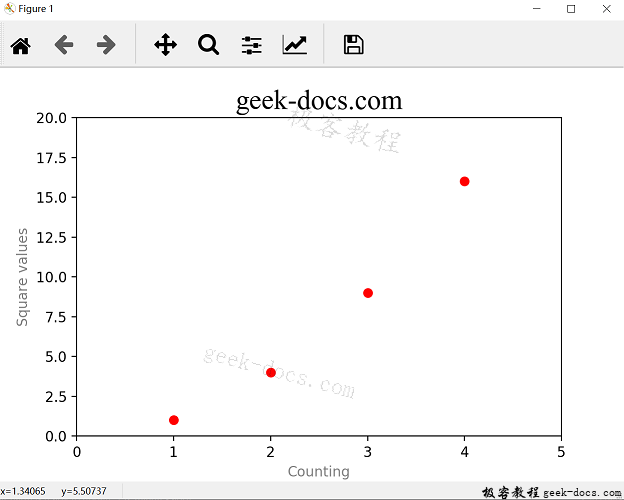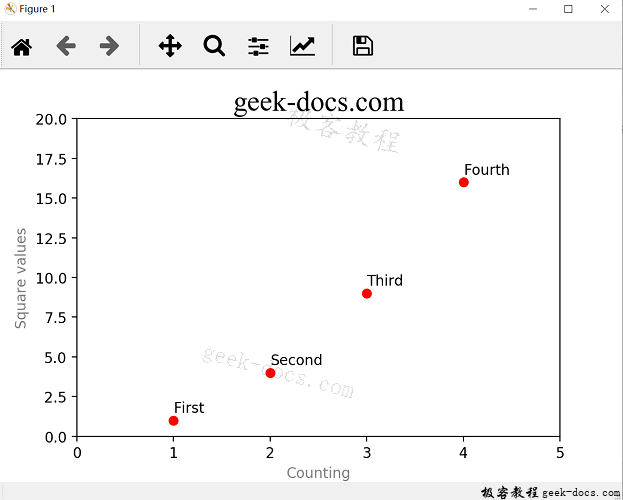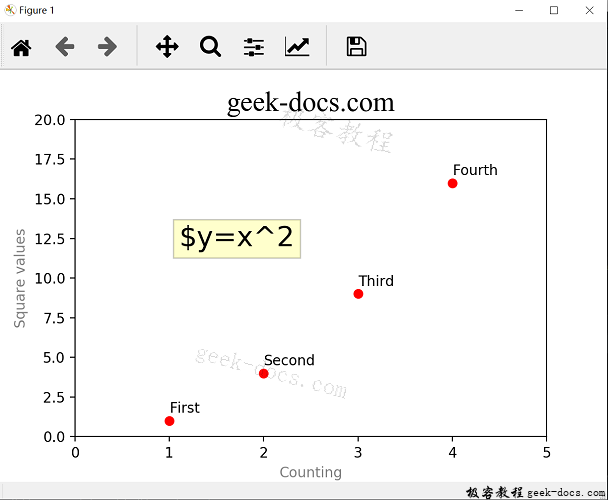# Matplotlib 添加文本

Matplotlib 添加文本，本文介绍如何为图表添加文字标签，极客教程前面章节介绍标题的添加方法，使用title()函数即可。另外xlabel()ylabel()函数专门用于添加轴标签，text()函数允许在图表任意位置添加文本。

## xlabel() 和 ylabel() 函数

import matplotlib.pyplot as plt

plt.axis([0,5, 0, 20])
plt.title("geek-docs.com")
plt.xlabel('Counting')
plt.ylabel('Square values')
plt.plot([1,2,3,4], [1,4,9,16], 'ro')
plt.show()import matplotlib.pyplot as plt

plt.axis([0,5, 0, 20])
plt.title("geek-docs.com", fontsize=20, fontname="Times New Roman")
plt.xlabel('Counting', color = 'gray')
plt.ylabel('Square values', color = 'gray')
plt.plot([1,2,3,4], [1,4,9,16], 'ro')
plt.show()## text() 函数

pyplot允许在图表任意位置添加文本，这个功能由text()函数来实现，函数定义如下:

text(x, y, s, fontdict=None, **kwargs)


text()前两个参数为标签在图形中位置的坐标，我们可以使用四个数据点的坐标作为各标签的坐标，如下所示：

import matplotlib.pyplot as plt

plt.axis([0,5, 0, 20])
plt.title("geek-docs.com", fontsize=20, fontname="Times New Roman")
plt.xlabel('Counting', color = 'gray')
plt.ylabel('Square values', color = 'gray')
plt.text(1, 1.5, 'First')
plt.text(2, 4.5, 'Second')
plt.text(3, 9.5, 'Third')
plt.text(4, 16.5, 'Fourth')
plt.plot([1,2,3,4], [1,4,9,16], 'ro')
plt.show()## LaTex表达式

Matplotlib 整合了LaTex表达式，支持在图表中插入数学表达式。将表达式内容置于两个$符号之间，可以在文本中添加Latex表达式。解释器会将符号之间的文本识别成LaTex表达式，把它们转换为数学表达式、公式、数学符号或希腊字母等。然后在图像中显示出来。通常，你需要在包含Latex表达式的字符串前添加r字符，表明它后面是原始文本，不能对其进行转义字符 现在我们使用关键字参数进一步丰富图形中的文本，添加描述图形各数据点趋势的公式，并为公式添加一个彩色边框。如下所示： import matplotlib.pyplot as plt plt.axis([0,5, 0, 20]) plt.title("geek-docs.com", fontsize=20, fontname="Times New Roman") plt.xlabel('Counting', color = 'gray') plt.ylabel('Square values', color = 'gray') plt.text(1, 1.5, 'First') plt.text(2, 4.5, 'Second') plt.text(3, 9.5, 'Third') plt.text(4, 16.5, 'Fourth') plt.text(1.1, 12, r'$y=x^2', fontsize=20, bbox={'facecolor':'yellow', 'alpha' : 0.2})
plt.plot([1,2,3,4], [1,4,9,16], 'ro')
plt.show()• 回顶